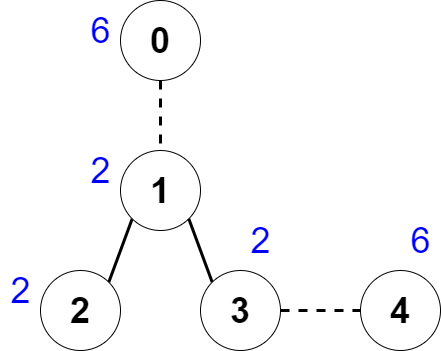2440. Create Components With Same Value
Hard
316
4

There is an undirected tree with `n` nodes labeled from `0` to `n - 1`.

You are given a 0-indexed integer array `nums` of length `n` where `nums[i]` represents the value of the `ith` node. You are also given a 2D integer array `edges` of length `n - 1` where `edges[i] = [ai, bi]` indicates that there is an edge between nodes `ai` and `bi` in the tree.

You are allowed to delete some edges, splitting the tree into multiple connected components. Let the value of a component be the sum of all `nums[i]` for which node `i` is in the component.

Return the maximum number of edges you can delete, such that every connected component in the tree has the same value.

Example 1:```Input: nums = [6,2,2,2,6], edges = [[0,1],[1,2],[1,3],[3,4]]
Output: 2
Explanation: The above figure shows how we can delete the edges [0,1] and [3,4]. The created components are nodes , [1,2,3] and . The sum of the values in each component equals 6. It can be proven that no better deletion exists, so the answer is 2.
```

Example 2:

```Input: nums = , edges = []
Output: 0
Explanation: There are no edges to be deleted.
```

Constraints:

• `1 <= n <= 2 * 104`
• `nums.length == n`
• `1 <= nums[i] <= 50`
• `edges.length == n - 1`
• `edges[i].length == 2`
• `0 <= edges[i], edges[i] <= n - 1`
• `edges` represents a valid tree.
Accepted
4.6K
Submissions
8.6K
Acceptance Rate
53.4%

Seen this question in a real interview before?
1/4
Yes
No

Discussion (0)

Related Topics